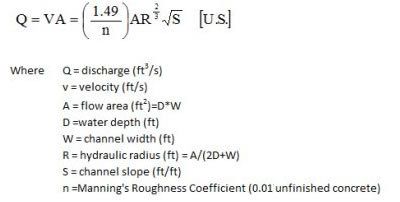# Discharge in a Roman Aqueduct (Application of Manning’s Equation)

The ancient Roman aqueducts consisted of partially filled channels above and below ground having rectangular cross section. The flow can be modeled as open channel flow using an equation called the Manning’s Equation.In this exercise you will calculate the velocity and discharge of water in the Marcia aqueduct channel in Aqueduct Park in Rome, Italy. The video below starts with Dr. Darius Arya introducing the aqueducts (behind him is the arcade of the combined Claudia and Ano Novus aqueducts in Aqueduct Park. Then Dr. Gary Robbins will be shown taking measurements in the Marcia channel. Take down the measurements and record them below.Â  Given the parameters recorded and those given below, using your calculator or a spreadsheet solve manning’s equation for the water velocity (ft/s) and dischargeÂ  (cubic ft/ second).Â  Â Be sure your parameter units conform to those in the equation shown above.

The distance from Aqueduct Park to the Roman Forum (center of ancient Roman government and religion) is 4.6 miles. Determine the time it would take for the water to travel from Aqueduct Park to the Forum. Insert your answer into the form below.

Using the discharge, determine how many gallons of water flowed to the city in a single day (recall there is 7.48 gallons/cubic foot of water).Â  Â Enter your answer in the form below and click submit.

Narration:
Dr. Gary Robbins, Dept. of Natural Resources and the Environment, U. Connecticut, Storrs, CT
Dr. Darius Arya, Director, American Institute for Roman Culture, Rome, Italy
Music:
Michael Levy, â€œOde to Ancient Romeâ€ (used with permission)
Video:
Gary Robbins and Michael Gemmell

### Mannings equation form

• W in Manning's Equation
• D in Manning's Equation
• S in Manning's Equations
• A in Manning's Equation = D*W
• R in Manning's Equation = A/(2D+W)
• V = Q/A
• Be sure to convert miles to feet ( 5280 feet/mile). Be sure to convert seconds to days (86400 seconds/day). Time = Distance/Velocity = 4.6*5280/(flow velocity (ft/s) *86400)
• gallons of water= Q*86400sec/day*7.48 gallons/cubic ft
• This field is for validation purposes and should be left unchanged.